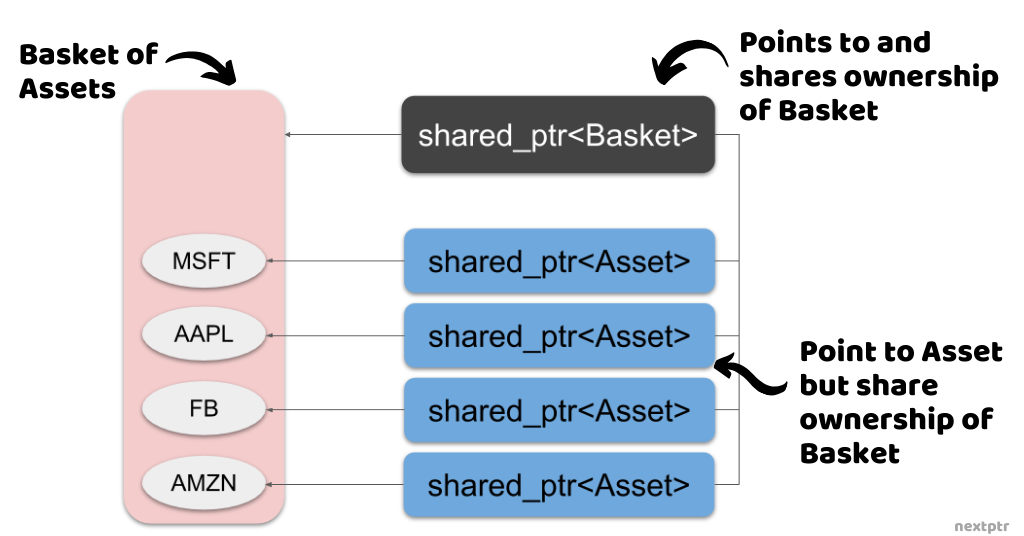# Aliasing constructed shared_ptr as key of map or set

## The Concept

Two std::shared_ptr instances can be compared with the defined relational operators for the std::shared_ptr<T> class (through the operator <=> since C++20). Therefore, std::shared_ptr can be used as keys in associative containers such as std::map, std::unordered_map, std::set, and std::unordered_set:

``````//Default comparison with std::less (<)
std::map<std::shared_ptr<std::string>, std::string> amap;

auto sps = std::make_shared<std::string>("Hello");

amap[sps] = "World"; //OK
``````

Note that the shared_ptr relational operators compare only the held raw pointers (the pointer returned by the get() method). So if two shared_ptrs are holding the same raw pointer, they would compare equal:

``````auto ip1 = std::make_shared<int>(10);
auto ip2 = ip1;

std::cout << (ip1 < ip2 ? "True" : "False"); //Always False
std::cout << (ip1 == ip2 ? "True" : "False"); //Always True
``````

However, a shared_ptr can hold a raw pointer that is different than the pointer to the managed object, which gets deleted when the reference count reaches zero. This use case of shared_ptr is quite peculiar and is done through the aliasing constructor of std::shared_ptr<`T`>:

``````template <class Y>
shared_ptr (const shared_ptr<Y>& r, T* ptr) noexcept;
``````

The aliasing constructor takes a shared_ptr (r above) with which the object ownership is shared, and an unrelated pointer (ptr above) that is held as the raw pointer and returned by the get() method. Therefore, a shared_ptr is capable of pointing at one object and managing the other. The common use of aliasing constructor is in creating a shared_ptr that holds a raw pointer to a part or member of an object but still manages the enclosing object. Here is an example:

``````struct Part {
//..
};

struct Whole {
Part p1, p2;
//...
};

std::shared_ptr<Part> foo() {
auto wp = std::make_shared<Whole>();

//Return std::shared_ptr<Part> using aliasing
return std::shared_ptr<Part>(wp, &wp->p1);

//The Whole object is not deleted on return
}

//call foo in a function
void bar() {
/*pp is a shared_ptr<Part> but manages a Whole object*/
auto pp = foo();
//'Whole' object is disposed of when this block ends
}
``````

In those cases where the held raw pointer is different than the managed pointer, it could be desirable to compare the managed pointers instead of the raw pointer. The std::owner_less function object essentially compares the managed pointers and can be used as a comparator with associative containers in place of the default std::less comparator. The std::owner_less merely calls the owner_before method of shared_ptr. Here is the comparison between std::less and std::owner_less:

``````//Create an alias for readability
using PartPtr = std::shared_ptr<Part>;

auto wp = std::make_shared<Whole>();

//Create std::shared_ptr<Part> using aliasing
auto pp1 = PartPtr(wp, &wp->p1);
auto pp2 = PartPtr(wp, &wp->p2);

/*Shows that pp1 and pp2 are compared as equal
using std::owner_less but not with std::less*/
std::cout << std::boolalpha
<< std::less<PartPtr>()(pp1, pp2) //true
<< std::less<PartPtr>()(pp2, pp1) //false
<< std::owner_less<PartPtr>()(pp1, pp2) //false
<< std::owner_less<PartPtr>()(pp2, pp1); //false

//create a set with default std::less comparator
std::set<PartPtr> pset;
//create a set with std::owner_less comparator
std::set<PartPtr,std::owner_less<PartPtr>> pownerset;

pset.insert(pp1); //inserts
pset.insert(pp2); //inserts

pownerset.insert(pp1); //inserts
pownerset.insert(pp2); //returns existing

std::cout << pset.size(); //2
std::cout << pownerset.size(); //1
``````

## An Example and A Question

Let's look at a more interesting example of using the aliasing constructor. In a financial application, we have an Asset class and a Basket class that contains a collection of Assets. Given a list of asset tickers (symbols e.g., IBM), the Basket class constructs and initializes its assets:

``````struct Asset {
std::string ticker;
//...more fields
};

//Load data from database and create assets
for(auto& t : tickers)
assets.push_back({t /*,more fields*/});
}
std::vector<Asset> assets;
};
``````

A function getBasketAssets (shown below) can fill a list of shared_ptr<`Asset`> for a given list of tickers. First, a Basket object is initialized that loads all the required assets. The Basket object is managed by a shared_ptr<`Basket`>. Then, multiple shared_ptr<`Asset`> instances are created using aliasing constructor from the shared_ptr<`Basket`> and pushed into a given list, as shown below:

``````void getBasketAssets(const std::vector<std::string>& tickers,
std::vector<std::shared_ptr<Asset>>& assets) {

for(auto& a : bp->assets) {
//Aliasing constructor
assets.push_back(std::shared_ptr<Asset>(bp, &a));
}
}
``````

Note that the Basket object is not disposed of when the getBasketAssets returns and the shared_ptr<`Basket`> is destroyed. The Basket object is deleted only when all the shared_ptr<`Asset`> are destroyed and the reference count reaches zero. The following illustration shows the relationship between the shared_ptr<`Asset`> and shared_ptr<`Basket`>:We call getBasketAssets a few times for different ticker lists and collect all the shared_ptr<`Asset`> instances in one vector<shared_ptr<Asset>>:

``````std::vector<std::shared_ptr<Asset>> allAssets;

``````

Suppose we want to get the counts of Asset objects from each Basket and do so by incrementing a counter for each shared_ptr<`Asset`> in a map, as shown:

``````using Comparator = _______; //Intentionally Omitted

std::map<std::shared_ptr<Asset>, size_t, Comparator> countMap;

for(auto& ap : allAssets)
countMap[ap]++;

for(auto& kvp : countMap)
std::cout << kvp.second << " ";
``````

The output of the above code depends on the Comparator we choose for the map, whose definition is intentionally omitted. As we created the three Baskets of 2,4 and 3 Assets above, we expect the above code to print "2 4 3" in no particular order. The code should not print all 1s ("1 1 1 1 1 1 1 1 1").

Select below a function object that satisfies our requirement as the Comparator (Check `Explanations` for details):